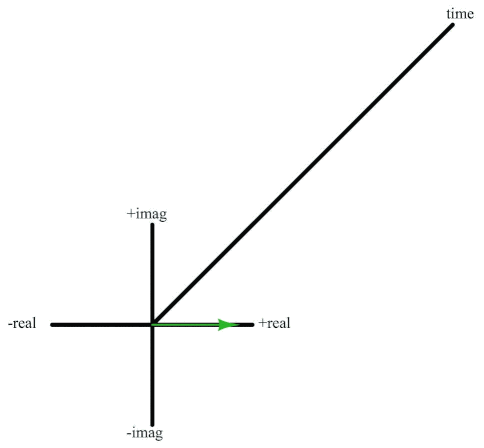Chapter More Principles of Industrial Instrumentation (Animated)

# Rotating Phasor in Three-dimensional Form

The following animation shows a rotating phasor in three-dimensional form. The phasor rotates in a complex plane (with real and imaginary axes), but travels linearly along the time axis. In doing so it traces a path that looks like a circle when viewed along the centerline (time axis) but looks like a sinusoidal wave when viewed from above or along the side.

[animation_rotating_phasor]

Euler’s Relation describes the phasor’s position in the complex plane:

$e^{j \omega t} = \cos \omega t + j \sin \omega t$

Where,

$$e$$ = Euler’s number (approximately equal to 2.718281828)

$$\omega$$ = Angular velocity, in radians per second

$$t$$ = Time, in seconds

$$\cos \omega t$$ = Horizontal projection of phasor (along a real number line) at time $$t$$

$$j \sin \omega t$$ = Vertical projection of phasor (along an imaginary number line) at time $$t$$

If you imagine the phasor’s length either growing or decaying exponentially over time, the result will be a spiral that either widens like a horn or shrinks like a funnel. This would be a visualization of a complex exponential, where the $$s$$ variable defines both the rate of growth/decay (the envelope of the spiral) and the angular velocity (the pitch of the spiral):

$e^{st} = e^{(\sigma + j\omega)t} = e^{\sigma t} e^{j \omega t}$

Where,

$$s$$ = Complex growth/decay rate and frequency (sec$$^{-1}$$)

$$\sigma$$ = $$1 \over \tau$$ = Real growth/decay rate (time constants per second, or sec$$^{-1}$$)

$$j \omega$$ = Imaginary frequency (radians per second, or sec$$^{-1}$$)

$$t$$ = Time (seconds)

The example of a unit-length rotating phasor is nothing more than a special case of the complex exponential, where $$\sigma = 0$$ (i.e. there is no growth or decay over time):

$e^{st} = e^{(0 + j\omega)t} = e^{0} e^{j \omega t} = e^{j \omega t}$#### Lessons in Industrial Automation

Published under the terms and conditions of the Creative Commons Attribution 4.0 International Public License